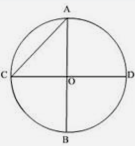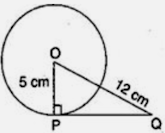# MCQ Questions for Class 10 Maths Chapter 10 Circles with Answers

Below you will find NCERT MCQ Questions for Class 10 Maths Chapter 10 Circles with Answers which is very helpful during the preparation of examinations. These MCQ Online Test are one marks questions which will give you overview of the chapter in no time. One should try to understand Class 10 MCQ Questions as it is based on latest exam pattern released by CBSE.

Also, students can check NCERT Solutions for Class 10 Maths Chapter 10 for improving their marks and have good understanding of the chapter.## Chapter 10 Circles MCQ Questions for Class 10 Maths with Answers

1. A tangent to a circle is a line that intersects the circle in
(a) Exactly one point
(b) 2 points
(c) 3 points
(d) 4 points
Solution (a) Exactly one point

2. At point A on a diameter AB of a circle of radius 10 cm, tangent XAY is drawn to the circle. The length of the chord CD parallel to XY at a distance 16 cm from A is
(a) 8 cm
(b) 10 cm
(c) 16 cm
(d) 18 cm
Solution (c) 16 cm

3. If TP and TQ are two tangents to a circle with centre O so that ZPOQ = 110°, then, ZPTQ is equal
to
(a) 60°
(b) 70°
(c) 80°
(d) 90°
Solution (b) 70°

4. Two parallel lines touch the circle at points A and B respectively. If area of the circle is 25 cm,2,, then AB is equal to
(a) 5 cm
(b) 7 cm
(c) 8 cm
(d) 10 cm
Solution (c) 8 cm

5. The maximum number of common tangents that can be drawn to two circles intersecting at two distinct point is
(a) 2
(b) 4
(c) 1
(d) 3
Solution (a) 2

6. In the given figure, length of BC is:(a) 9 cm
(b) 10 cm
(c) 13 cm
(d) 21 cm
Solution (c) 13 cm

7. A tangent PQ at a point P of a circle of radius 5 cm meets a line through the centre O at a point Q so that OQ = 12 cm. Length PQ is :
(a) √119 cm
(b) 13 cm
(c) 12 cm
(d) 8.5 cm
Solution (a) √119 cm

8. The length of tangent PQ, from an external point P is 24 cm. If the distance of the point P from the centre is 25 cm, then the diameter of the circle is(a) 7 cm
(b) 14 cm.
(c) 15 cm.
(d) 12 cm
Solution (b) 14 cm.

9. PQ is a tangent drawn from a point P to a circle with centre O and QOR is a diameter of the circle such that ∠POR = 120°, then ∠OPQ is
(a) 60°
(b) 45°
(c) 30°
(d) 90°
Solution (c) 30°

10. A circle touches x-axis at A and y-axis at B. If O is origin and OA = 5 units, then diameter of the circle is
(a) 8 units
(b) 10 units
(c) 10 √2 units
(d) 8 √2 units
Solution (b) 10 units

11. In a circle with centre O, AB and CD are two diameters perpendicular to each other. The length of chord AC is(a) 1/√2 AB
(b) 1/2 AB
(c) √2 AB
(d) 2 AB
Solution (a) 1/√2 AB

12. The length of the tangent from a point A at a circle, of radius 3 cm, is 4 cm. The distance of A from the centre of the circle is
(a) √7 cm
(b) 7cm
(c) 5 cm
(d) 25 cm
Solution (c) 5 cm

13. Two parallel lines touch the circle at points A and B respectively, If area of the circle is 25πcm2,, then AB is equal to
(a) 5 cm
(b) 8 cm
(c) 10 cm
(d) 25 cm
Solution (c) 10 cm

14. If four sides of a quadrilateral ABCD are tangential to a circle, then
(a) AC + AD = BD + CD
(b) AB + CD = BC + AD
(c) AB + CD = AC + BC
(d) AC + AD = BC + DB
Solution (b) AB + CD = BC + AD

15. A tangent PQ at a point P o a circle of radius 5 cm meets a line through the centre O at point Q, so that OQ = 12 cm. find the length PQ.(a) √113 cm
(b) 13 cm
(c) √119 cm
(d) 26 cm
Solution (c) √119 cm

16. If the angle between two radii of a circle is 100°, then angle between the tangents at the ends of those radii is:​
(a) 80°
(b) 90°
(c) 60°
(d) 50°
Solution (a) 80°

17. A point P is 25 cm from the centre of a circle. The radius of the circle is 7 cm and length of the tangent drawn from P to the circle is x cm. The value of x =
(a) 20 cm
(b) 24 cm
(c) 18 cm
(d) 12 cm
Solution (b) 24 cm

18. A circle is inscribed in a ΔABC having AB = 10cm, BC = 12cm and CA = 8cm and touching these sides at D, E, F respectively. The lengths of AD, BE and CF will be(a) AD = 4cm, BE = 6cm, CF = 8cm
(b) AD = 5cm, BE = 9cm, CF = 4cm
(c) AD = 3cm, BE = 7cm, CF = 5cm
(d) AD = 2cm, BE = 6cm, CF = 7cm
Solution (c) AD = 3cm, BE = 7cm, CF = 5cm

19. A point P is 10 cm from the centre of a circle. The length of the tangent drawn from P to the circle is 8 cm. The radius of the circle is equal to
(a) 4 cm
(b) 5 cm
(c) 6 cm
(d) None of these.
Solution (c) 6 cm

20. At one end of a diameter PQ of a circle of radius 5 cm, tangent XPYis drawn to the circle. The length of chord AB parallel to XY and at a distance of 8 cm from P is
(a) 6 cm.
(b) 5 cm.
(c) 7 cm.
(d) 8 cm
Solution (d) 8 cm

21. If angle between two radii of a circle is 130°, the angle between the tangents at the ends of the radii is
(a) 90°
(b) 50°
(c) 70°
(d) 40°
Solution (b) 50°